# contrast with highlights roll-off

Just for fun here a way to automatically recover the highlights from a simple contrast formula

contrast=pow((input/fulcrum),cont)*fulcrum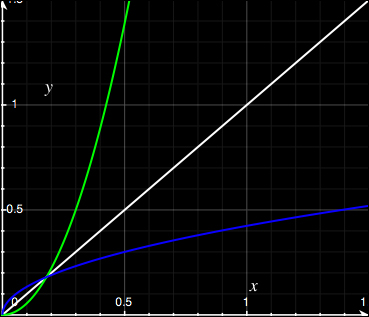https://compositormathematic.wordpress.com/

Basically for this task Reinhard tonemapping operator is used and scaled

Test image

image with contrast

``````fulcrum=0.15
cont=1.8
#contrast
c = pow((input/fulcrum),cont)*fulcrum
``````

image with contrast and smooth roll-off of the highlights to 1.0

``````fulcrum=0.15
cont=1.8

#contrast
c = pow((input/fulcrum),cont)*fulcrum

#find how much the max value (1.0) is raised after contrast
max_value= pow((1/fulcrum),cont)*fulcrum

#find how much the fulcrum value is darkened after tonemapping
fulcrum1=(fulcrum*(1+ (fulcrum /(max_value*max_value))))/(1+fulcrum)

#tonemapping
c1 = (c*(1+ (c /(max_value*max_value))))/(1+c)

#levels
c1 = ((c1 - fulcrum1) / (1 - fulcrum1))  * (1 - fulcrum) + fulcrum

#contrast with highlights roll off
output= if c <= fulcrum  c else c1
``````

formulas used

``````contrast=pow((input/fulcrum),cont)*fulcrum

reinhard=(input*(1+ (input /(max_value*max_value))))/(1+input)

levels = ((input - min_in) / (max_in - min_in))  * (max_out - min_out) + min_out
``````

In theory is possible to adapt this algo to work within a user defined range, for example in 0-1 range for sdr or 0-10 range for hdr10

1 Like

Just for fun, I tried to match your statistics (mean and standard deviation) and applied my 2-curve filter (brightness-contrast) that I use when I am lazy. The contrast curve part of the command certainly reverses the roll-off!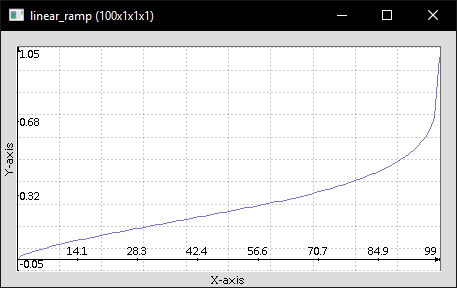Interesting, thanks. There seems to be a second-order discontinuity at fulcrum, where the two curves join.

1 Like

I see, that’s badI guess it would be better to just invert the image and use the same contrast formula for the highlightsIt looks like ASC CDL formula less offset plus fulcrum.
Or to agriggion ART formula less offset.
see https://www.geogebra.org/geometry/zbc3k7pz

1 Like

If we want a curve that passes through (0,0) and (1,1) and a fulcrum (F,F) where 0<F<1, with a contrast G at (F,F), one way is to join these two curves:

``````Where v <= F:
v' = (0.5*v+0.5*F*pow(v/F,G))

Where v >= F:
v' = 1-(0.5*(1-v)+0.5*(1-F)*pow((1-v)/(1-F),G))``````

We have first and second order continuity.

The gradient dv’/dv at (F,F) is (G+1)/2.

Using ImageMagick, Windows BAT syntax:

``````set F=0.15
set G=10

set FORM=^
u^<%F%?^
(0.5*u+0.5*%F%*pow(u/%F%,%G%)):^
1-(0.5*(1-u)+0.5*(1-%F%)*pow((1-u)/(1-%F%),%G%))

set OUTFILE=fulcrum_%G:.=_%.png

%IM%convert -size 1x256 gradient: -rotate 90 ^
-fx "%FORM%" ^
%OUTFILE%

call %PICTBAT%graph1d %OUTFILE%``````

In our situation, we want v’<v at 0<v<F, and v’>v at F<v<1, so we set G >= 1.

Examples at F=0.15, and G=1.5, 2, 3 and 10: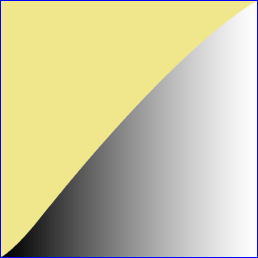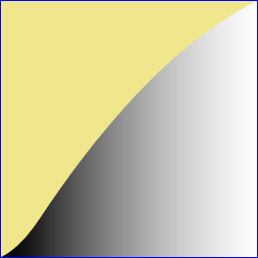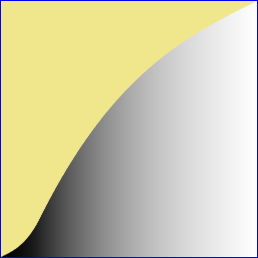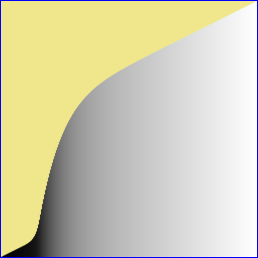1 Like

Thanks @snibgo !

Could this formula be used without introducing artifacts in your opinion ?

``````Where v <= F:
v' = (F*pow(v/F,G))

Where v >= F:
v' = 1-((1-F)*pow((1-v)/(1-F),G))``````

Yes.

EDIT to add: This transfer function has zero gradient at the top and bottom, so beware of crushing shadows and highlights. For example, F=0.15 and G=2 (comparable to the F=0.15 G=3 example above):That is what I use for my contrast curve. My lazy command selects the average value as the fulcrum, which is unsuitable for this scenario.

1 Like

Is it used in your soft light blending mode?

No and it isn’t available to the public. If you want to know what it does currently, it pivots at the average intensity and arrives at the user specified standard deviation. Been thinking of freeing up the pivot to allow more possibilities. As indicated above, the curve is on the rounded side; thus making the roll-off of both the shadows and highlights excessive.

It is a trade off really. Here is a comparison between the curve in post 6 and ours; fulcrum at average intensity and standard deviation set to roughly converge on 0.3.

Ours

His

Blend (average)

1 Like